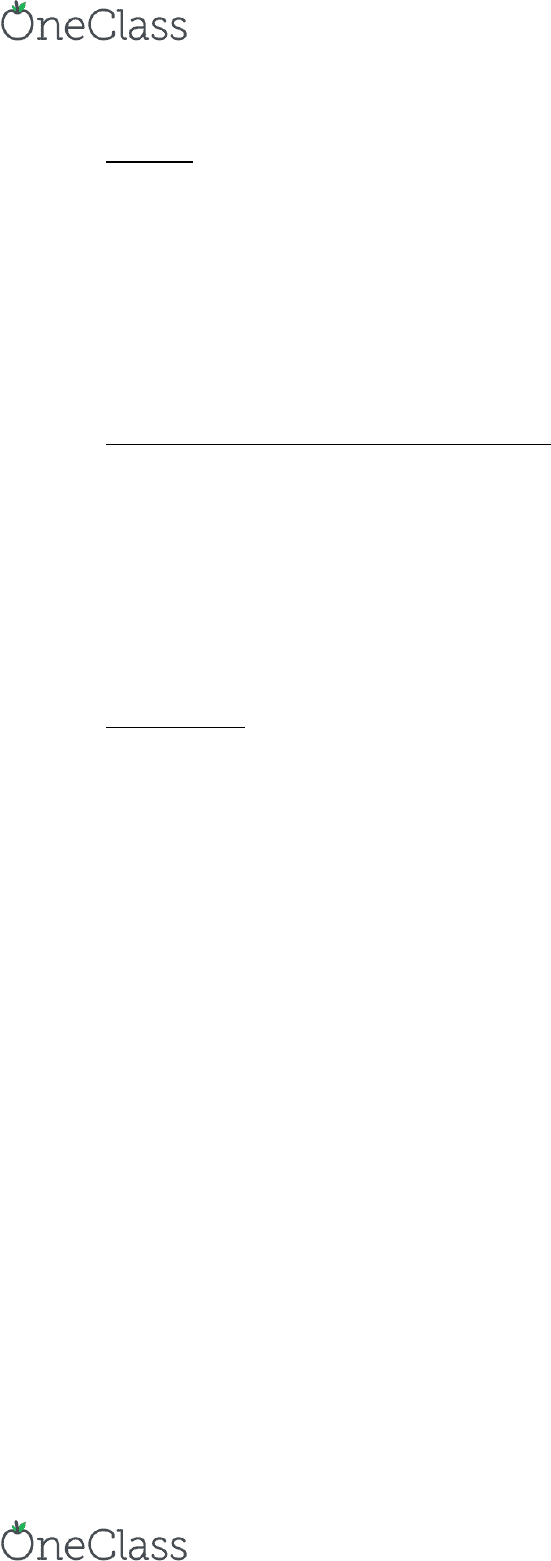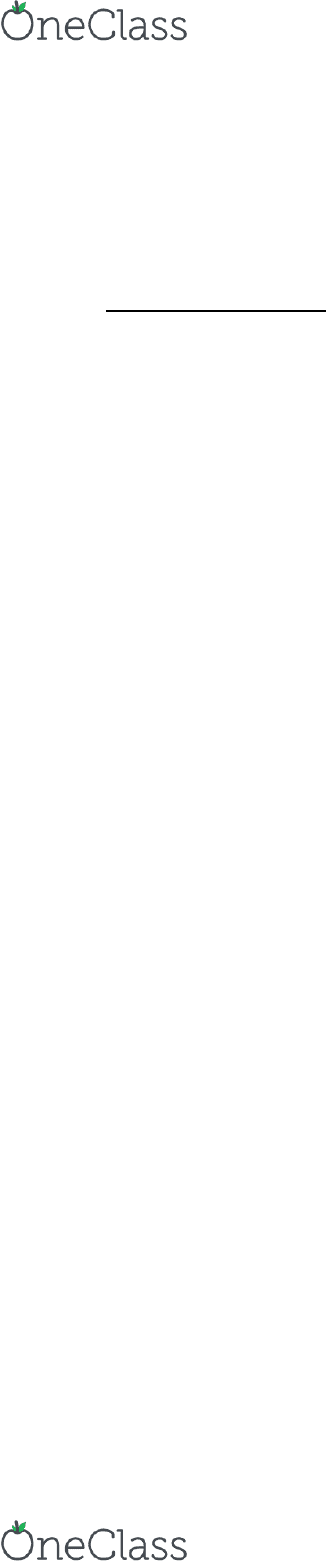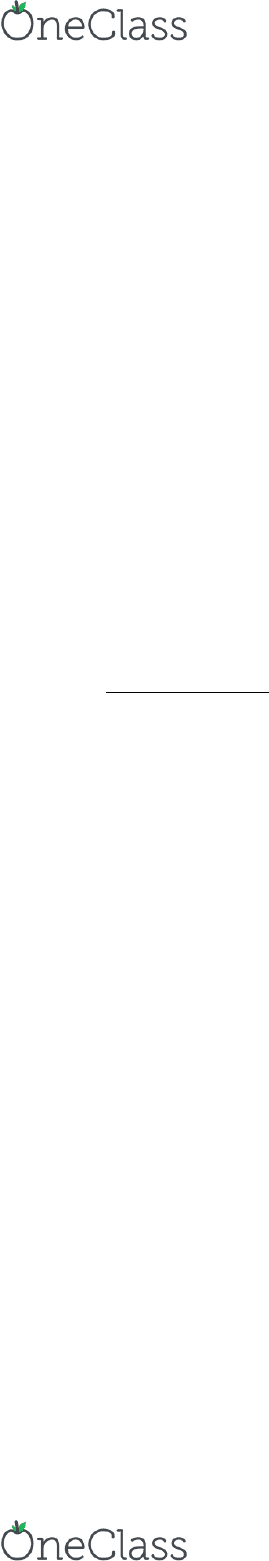Class Notes (1,100,000)
CA (650,000)
SFU (10,000)
BUS (1,000)
BUS 207 (20)
Lecture 8

# BUS 207 Lecture Notes - Lecture 8: Strategic Dominance, Mattress, Bertrand Competition

Department
Course Code
BUS 207
Professor
Karen Ruckman
Lecture
8

This preview shows pages 1-3. to view the full 9 pages of the document.Oligopoly
Market structure where there is a relatively small # of firms competing in a market & there are
some entrance barriers
Key feature: firms recognize their mutual interdependence
o Know their decisions will affect their rivals & vice versa
o competitors must determine how rival will respond to their actions
o This problem = realized by the game theory
Models that Recognize Interdependence of D-M:
Cournot
o 2 firms make simultaneous decisions about how much of a homogenous G to produce
Bertrand
o 2 firms make simultaneous decisions about the price of a homogenous G
Stackelberg
o 2 firms make sequential decisions about how much of a homogenous G to produce
Cournot Model
Assumptions:
o Equal size w/same costs
TC1 = TC2 = cq1 = cq2
2 firms of equal size, same costs/G produced (homogenous; substitutable)
o Homogenous products
E.g. farmers, vaccinations, anything grown/takes long to manufacture
o Market D curve is downward sloping
P = a Q = a (q1 + q2) = a q1 q2
Each firm knows the shape of the market D curve can predict P based on
different total industry Q
o Simultaneous choice of output
o Each firm chooses output by choosing the best response to rival actions
Chooses w/o knowing what the other has chosen
Problem = solved by: assuming each firm chooses profit maximizing Q by choosing the best
response to rival actions
Each firm maximizes profits based on an assumed Q that the other firm will produce:
o Fi ’s pofit aiizig 1:
TR = p*q = (a q1 q2) * q1
MR = a 2q1 q2
C = c*q1
MC = c
MR = MC
a 2q1 q2 = c
find more resources at oneclass.com
find more resources at oneclass.com

Only pages 1-3 are available for preview. Some parts have been intentionally blurred.q1 = (a - q2 c)/2
o Fi ’s pofit aiizig 2:
q2 = q1 = q1 = (a q1 c)/2
Graphs and Calculations
Firm 1 current sees itself as a monopoly but then assumes Firm 2 will produce the same Q
o must share industry (market) D so DF1 and q1 and MR1
Reatio f’s slope doads / he 2 = 0, F1has entire market
o As q1 , q2
But, q1 by less than what q2 produces
q2 produces more than what q1 by
o If q2 = 0, then q1 = (a c)/2
o If q2 = 0, then q2 = (a c)/2
o Meaning, q1 goes down by q2/2
Equilibrium Q where both firms maximize profits = q2 = 1/3(a-c)
o Occurs where neither player has incentive to deviate b/c chosen a strategy that
maximizes profits, given the strategy of the other firm (Nash Equi)
Market:
o Q = q1 + q2
= 2 * 1/3(a c)
o P = a Q
= a - 2 * 1/3(a c)
= 1/3a + 2/3c
Recall that relative to PC firm, M will restrict Q to P
Given the same D & C structures, Cournot duopoly = P/Q combo that is between a PC & M
o W/o collusion, restricts Q to level that P above competitive P allows firms to earn
above-formal profits
But profit as high as M profit
Assuming: P = a c and Tc = c*Q, profit-maximizing Qs:
o PC: Q = a c
b/c c = a Q
Solve for Q
o M: Q = 1/2(a c)
b/c TR = a Q so MR = a 2Q
MC = c
MR = MC a 2Q = c
Solve for Q
o CD: Q = 2/3(a c)
Copaig the fatios fo PC, M, ad CD, PC > CD > M hee h CD is i etee
Comparing PC & CD: (a c) vs 2/3(a c)
o In general, the output of an n-firm industry behaving as a CD is:
Q = (n/n+1)(a - c)
find more resources at oneclass.com
find more resources at oneclass.com

Only pages 1-3 are available for preview. Some parts have been intentionally blurred.o Industry Q & P thus depend on the number of firms more firms = more firm output
requires to profit maximize and P
So, CD will restrict output below competitive level & will earn above-normal
profit
Also notice how as n gets larger (approaches infinity), n/n+1 approaches 1 aka
gets closer to an industry that behaves as a PC b/c lose oligopoly power
Profit margins in crease as more firms enter the market
o (P C)/profit margin = H
H = Herfindahl index sum of squared market share of each firm in industry
Measure of industry concentration
If all firms had equal market share, 1/n = H
What H tells us:
H is large (1) then the market is more concentrated and margins are
high M
H is small (0) then the market is less concentrated and margins are low
PC
Bertrand Duopoly
Firms produce a homogenous product but compete on P
o Equi P & industry Q = same as under PC
o Outcome occurs b/c best response to any P chosen by competitor is a lower P let’s
you take the whole market
So, every firm will choose the lowest possible P (according to MC)
o In the end, each firm ears 0 profit b/c keep over/under-cutting e/o equalizes profit and
losses to 0
Limits of Bertrand:
o When firms = capacity-constrained (limited in size) b/c steal the whole market by
under-cutting rivals
P competition is limited
Limits effectiveness b/c steal the whole market (as the model
assumes)
Meanwhile, excess capacity = encourages P competition
E.g. seasons for vacationing: peak or quiet
@ peak = compete w/installed capacity b/c cannot serve all of market
(travellers)
@ quiet = airlines have planes that take off even when empty; have to
sell seats @ lower P
o When products = differentiated b/ fis ≠ steal the etie aket  ↓ P sie soe
customers will prefer to pay a higher P for a product they prefer
So, product differentiation = market power for each firm and P
competition
fully capture the nature of P comp
find more resources at oneclass.com
find more resources at oneclass.com